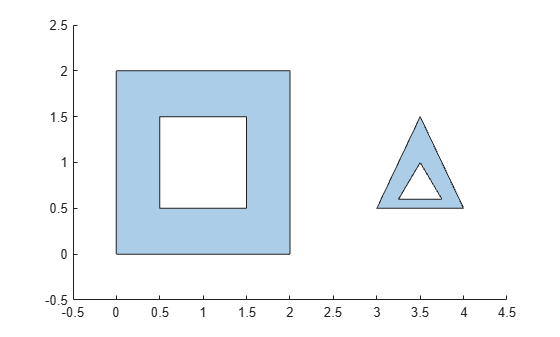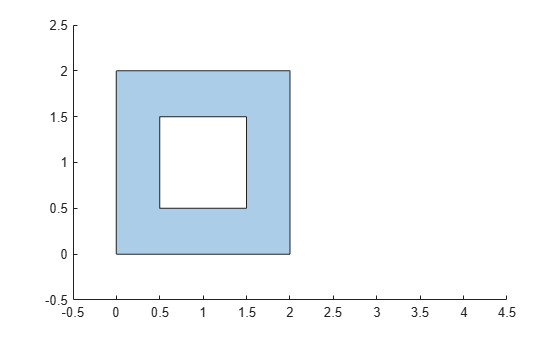# regions

Access `polyshape` regions

## Syntax

``polyout = regions(polyin)``

## Description

example

````polyout = regions(polyin)` returns a vector of `polyshape` objects whose elements are the solid regions of the input `polyshape`.```

## Examples

collapse all

Compute a polygon with two solid regions. Then, create a vector whose elements are a single region of the polygon.

```P = [0 0; 0 2; 2 2; 2 0; NaN NaN; 0.5 0.5; 0.5 1.5; 1.5 1.5; 1.5 0.5; NaN NaN; 3 0.5; 3.5 1.5; 4 0.5; NaN NaN; 3.25 0.6; 3.75 0.6; 3.5 1]; polyin = polyshape(P); plot(polyin) xlim([-0.5 4.5]) ylim([-0.5 2.5])````polyout = regions(polyin)`
```polyout = 2x1 polyshape array with properties: Vertices NumRegions NumHoles ```
```plot(polyout(1)) xlim([-0.5 4.5]) ylim([-0.5 2.5])``````plot(polyout(2)) xlim([-0.5 4.5]) ylim([-0.5 2.5])```## Input Arguments

collapse all

Input `polyshape`, specified as a scalar.

Data Types: `polyshape`